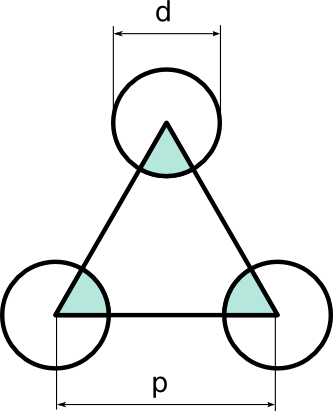# The open area for triangular pitch perforated sheets

For heat exchanger tube plates and for sieve trays in columns, the triangular pitch arrangement is common. You can find ready-to-use formulas on the web to calculate the open area of a perforated sheets, such as this one on the website of RMIG:But since we do not trust Google’s I’m Feeling Lucky suggestions out-of-the-box, and additionally using R for a diameter is disturbing, here is the derivation (using lowercase d for the hole diameter and p for the pitch, as it should be):= (d^2*π/8) / (p^2*sqrt(3)/4) = d^2/p^2 * (π/2/sqrt(3)).

The constant π/2/sqrt(3) = 0.906899682119 so Q.E.D.